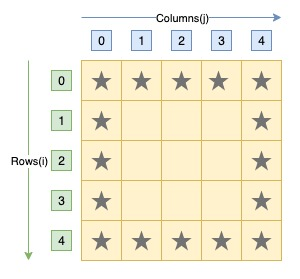# C Program to Print Hollow Square Star Pattern

Here is a C program to print outline or boundary of a square pattern by star character using loop. For a hollow square star pattern of side 5 stars. Program's output should be:#### Required Knowledge

Algorithm to print hollow square star pattern using loop
The algorithm of this pattern is similar to square star pattern except here we have to print stars of first and last rows and columns. At non-boundary position, we will only print a space character.
• Take the number of stars in each side of square as input from user using scanf function. Let it be N.
• We will use two for loops to print square star pattern.
• Outer for loop will iterate N times. In one iteration, it will print one row of pattern.
• Inside inner for loop, we will add a if statement check to find the boundary positions of the pattern and print star (*) accordingly.

Here is the matrix representation of the hollow square star pattern. The row numbers are represented by i whereas column numbers are represented by j.## C program to print hollow square star pattern

```#include<stdio.h>

int main(){
int side, i, j;

printf("Enter side of square\n");
scanf("%d", &side);

/* Row iterator for loop */
for(i = 0; i < side; i++){
/* Column iterator for loop */
for(j = 0; j < side; j++){
/* Check if currely position is a boundary position */
if(i==0 || i==side-1 || j==0 || j==side-1)
printf("*");
else
printf(" ");
}
printf("\n");
}
return 0;
}
```
Output
```Enter side of square
5
*****
*   *
*   *
*   *
*****
```

Related Topics
 C program hollow rectangle pattern C program hollow diamond star pattern C program hollow pyramid star pattern C program square star pattern C program right triangle star pattern C program pyramid star pattern C program heart shape star pattern C program rhombus star pattern C program natural number triangle pattern List of all C pattern programs